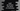# Swift program to get all keys and values of a dictionarySwift dictionary is used to hold key-value pairs. Using a key, we can get the value of a specific dictionary key-value pair. Similarly, we can also modify or delete dictionary values using its key.

This tutorial will show you how to get all keys and values of a swift dictionary. Swift dictionary provides two instance properties keys and values that returns a collection containing all keys or values.

## keys :

This instance property is defined as below :

``var keys: Dictionary<Key, Value>.Keys { get }``

It returns one collection containing all the keys of the dictionary.

### Example :

``````var given_dict = ["one": 1, "two": 2, "three": 3]

print(given_dict.keys)``````

It will print :

``["two", "one", "three"]``

## values :

This instance property is defined as below :

``var values: Dictionary<Key, Value>.Values { get set }``

It returns one collection containing all the values of the dictionary.

### Example :

``````var given_dict = ["one": 1, "two": 2, "three": 3]

print(given_dict.values)``````

It will print:

``[1, 3, 2]``